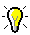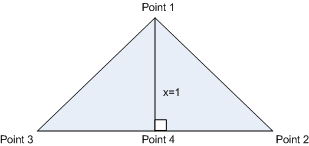﻿ route
SiteWatch API Help

# route# routeA method to set a route from one map location to another map location.

Parameter

Value

fromX: number

isn93 x-coordinate of start location.

fromY: number

isn93 y-coordinate of start location.

toX: number

isn93 x-coordinate of end location.

toY: number

isn93 y-coordinate of end location.

searchDistance: integer (optional)

Radius of area from point in meters to the street.

simplify: integer (optional)

Line simplicity in meters (0 = nothing is simple, n = By how much can the line from x be slided so that points can be removed).

Simplification removes extraneous bends and small intrusions and extrusions from a line or polygon boundary without destroying its essential shape. It is efficient for data compression and for eliminating redundant details.

The line that results may contain sharp angles and spikes that reduce the cartographic quality of the line.

Use for small amounts of data reduction or compression and when high cartographic quality is not needed.

function: name

An asynchronous JavaScript function that implements the logic when the function call returns.

scope: name (optional)

Owner of the current object.Image below to the right: Simplify=1 then Point 1 is not removed from the route. Simplify=2 then the distance x = 1 from the line (from imaginary Point 4) between Point 2 and 3 to the Point 1 is < 2 then Point 1 is removed from the route.   Image below to the left: Yellow line is more simplified than the green line and thus results in less detail of the route.Example:

function rResult(map, res)

{

var r = res.OK; //Search success (true) or failure (false).

res.data.points,

{ color: "blue", size: 3 });

var dir = res.data.direction;

var b = dir.b; //b = bearing (B = straight ahead, H = to the right, V = to the left).

var n = dir.n; //n = streetname.

var x = dir.p.x; //x-coordinate.

var y = dir.p.y; //y-coordinate.

var d = dir.d; //distance in meters.

var t = dir.t; //time in minutes.

var tt = res.data.time; //Total time in minutes.

var td = res.data.distance; //Total distance length in meters.

}

map.route(358455, 407655, 358852, 407286, 100, 0, rResult, this); //Short.

Or

map.route(358455, 407655, 540637, 575896, 100, 2, rResult, this); //Long.